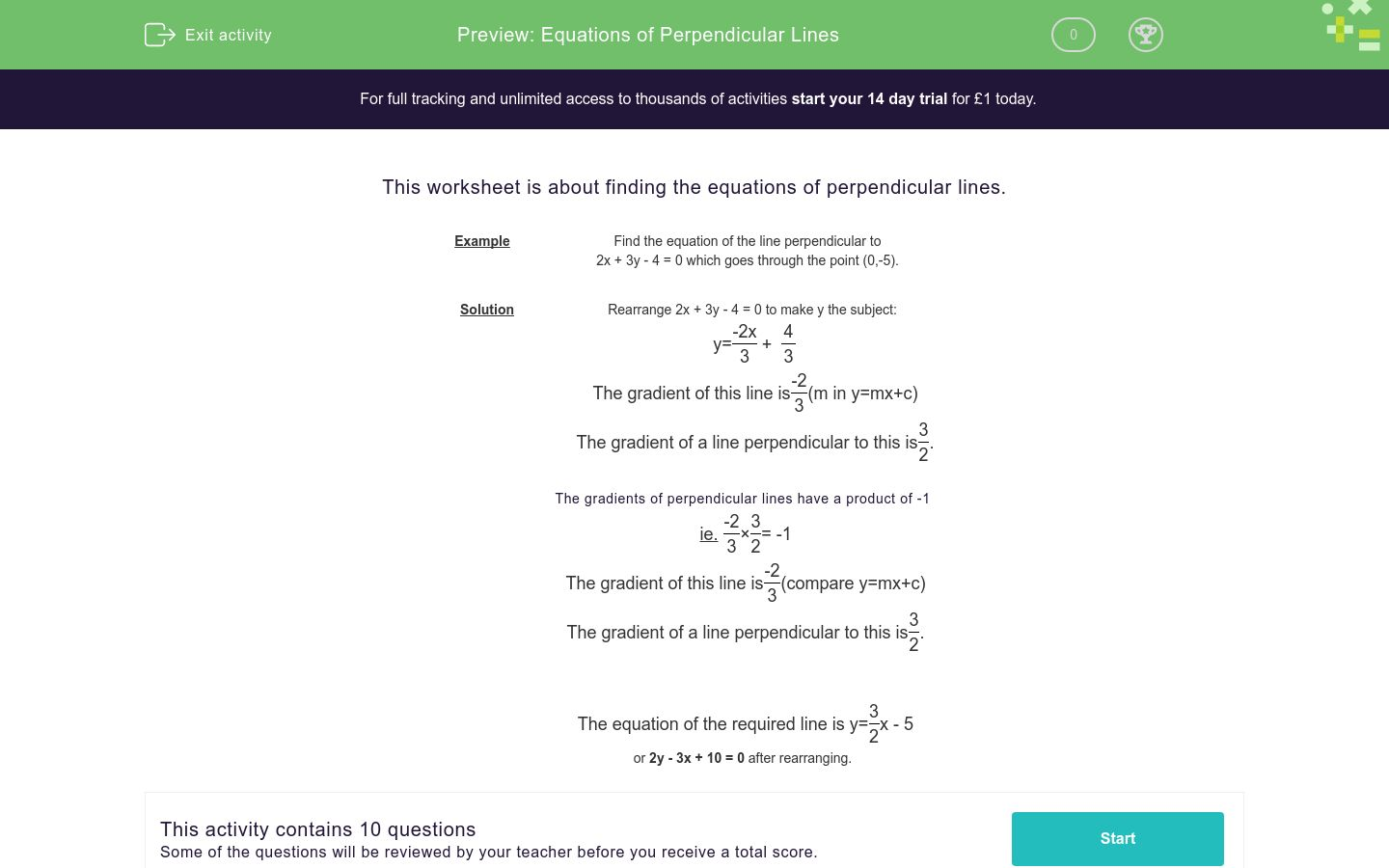# Equations of Perpendicular Lines

In this worksheet, students find the equations of perpendicular lines.Key stage:  KS 4

Curriculum topic:  Algebra

Curriculum subtopic:  Find the Equation of a Line

Difficulty level:### QUESTION 1 of 10

This worksheet is about finding the equations of perpendicular lines.

 Example Find the equation of the line perpendicular to 2x + 3y - 4 = 0 which goes through the point (0,-5).

Solution Rearrange 2x + 3y - 4 = 0 to make y the subject:
y=
 -2x 3
+
 4 3
The gradient of this line is
 -2 3
(m in y=mx+c)
The gradient of a line perpendicular to this is
 3 2
.

The gradients of perpendicular lines have a product of -1

ie.
 -2 3
×
 3 2
= -1
The gradient of this line is
 -2 3
(compare y=mx+c)
The gradient of a line perpendicular to this is
 3 2
.

The equation of the required line is y=
 3 2
x - 5
or 2y - 3x + 10 = 0 after rearranging.

Find the equation of the line perpendicular to -6x+2y-4=0 which passes through (0,2)

Find the equation of the line perpendicular to -6x+2y-4=0 which passes through (0,4)

Find the equation of the line perpendicular to x-4y-7=0 which passes through (0,6)

Find the equation of the line perpendicular to -4x-8y+3=0 which passes through (0,4)

Find the equation of the line perpendicular to 3x+6y-5=0 which passes through (0,2)

Find the equation of the line perpendicular to 7x+y+4=0 which passes through (0,-10)

Find the equation of the line perpendicular to 9x+10y+7=0 which passes through (0, 8)

Find the equation of the line perpendicular to 9x-3y-10=0 which passes through (0, -6)

Find the equation of the line perpendicular to 2x+9y+10=0 which passes through (0, 5)

Find the equation of the line perpendicular to 2x+9y+10=0 which passes through (1, 5)

• Question 1

Find the equation of the line perpendicular to -6x+2y-4=0 which passes through (0,2)

EDDIE SAYS
-3y-x+6=0
• Question 2

Find the equation of the line perpendicular to -6x+2y-4=0 which passes through (0,4)

EDDIE SAYS
-3y-x+12=0
• Question 3

Find the equation of the line perpendicular to x-4y-7=0 which passes through (0,6)

EDDIE SAYS
y+4x-6=0
• Question 4

Find the equation of the line perpendicular to -4x-8y+3=0 which passes through (0,4)

EDDIE SAYS
-y+2x+4=0
• Question 5

Find the equation of the line perpendicular to 3x+6y-5=0 which passes through (0,2)

EDDIE SAYS
y-2x-2=0
• Question 6

Find the equation of the line perpendicular to 7x+y+4=0 which passes through (0,-10)

EDDIE SAYS
7y-x+70=0
• Question 7

Find the equation of the line perpendicular to 9x+10y+7=0 which passes through (0, 8)

EDDIE SAYS
9y-10x-72=0
• Question 8

Find the equation of the line perpendicular to 9x-3y-10=0 which passes through (0, -6)

EDDIE SAYS
9y+3x+54=0
• Question 9

Find the equation of the line perpendicular to 2x+9y+10=0 which passes through (0, 5)

EDDIE SAYS
2y-9x-10=0
• Question 10

Find the equation of the line perpendicular to 2x+9y+10=0 which passes through (1, 5)